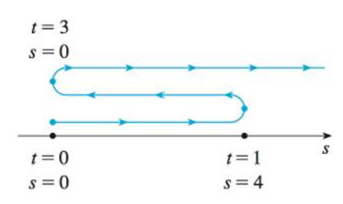Chapter 3.7, Problem 3E### Single Variable Calculus: Early Tr...

8th Edition
James Stewart
ISBN: 9781305270343

#### Solutions

Chapter
Section### Single Variable Calculus: Early Tr...

8th Edition
James Stewart
ISBN: 9781305270343
Textbook Problem

# A particle moves according to a law of motion s = f(t), t ≥ 0, where t is measured in seconds and s in feet.(a) Find the velocity at time t.(b) What is the velocity after 1 second?(c) When is the particle at rest?(d) When is the particle moving in the positive direction?(e) Find the total distance traveled during the first 6 seconds.(f) Draw a diagram like Figure 2 to illustrate the motion of the particle.(g) Find the acceleration at time t and after 1 second.(h) Graph the position, velocity, and acceleration functions for 0 ≤ t ≤ 6.(i) When is the particle speeding up? When is it slowing down?FIGURE 2f(t) = sin(πt/2)

(a)

To determine

To find: The velocity at time t.

Explanation

Given:

The given equation is as below.

s=f(t)=sin(πt2) (1)

Calculation:

Calculate the velocity at time t.

Differentiate the equation (1) with respect to time.

v(t)=f'(t)=cos(πt2)

(b)

To determine

To find: The velocity after 1 second.

(c)

To determine

To find: The time when particle at rest.

(d)

To determine

To find: The particle moving in the positive direction.

(e)

To determine

To find: The total distance traveled during the first 6 seconds.

(f)

To determine

To find: The diagram to illustrate the motion of the particle.

(g)

To determine

To find: The acceleration at time t and after 1 second.

(h)

To determine

To sketch: The graph the position, velocity, and acceleration function for 0t6.

(i)

To determine

To find: The time when the particle is speeding up and slowing down.

### Still sussing out bartleby?

Check out a sample textbook solution.

See a sample solution

#### The Solution to Your Study Problems

Bartleby provides explanations to thousands of textbook problems written by our experts, many with advanced degrees!

Get Started

#### Find more solutions based on key concepts